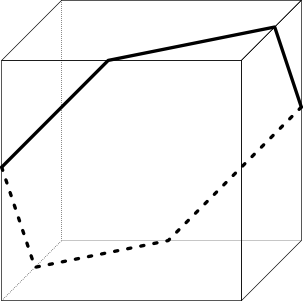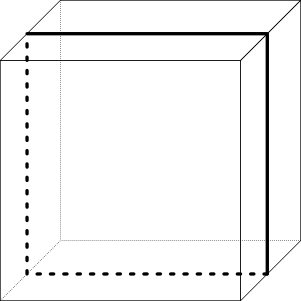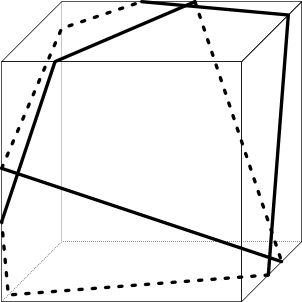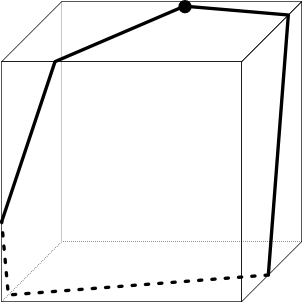시간 제한 메모리 제한 제출 정답 맞은 사람 정답 비율
2 초 256 MB 0 0 0 0.000%

## 문제

You want to wrap a cubic box using a ribbon. You tie the ribbon to form a cycle. In other words, the ribbon must be a closed curve on the surface of the box. The ribbon must be stretched: it must be straight on a surface, and when it crosses an edge, the two angles shown below must be the same. The ribbon is not allowed to pass through a corner.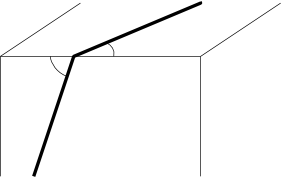Also, when the box is placed in three-dimensional space with a Cartesian coordinate system such that the edges are parallel to the coordinate axes, there must be a part of the ribbon that is parallel to the vector $(a, b, 0)$.

The side lengths of the box are $1$. Compute the minimum possible length of the ribbon. You can assume that the size of the knot is infinitely small, but the ribbon must be stretched even at the position of the knot (see the note below).

## 입력

You are given two integers $a$ and $b$ on a single line ($0 \le a, b \le 10^{18}$, $(a, b) \ne (0, 0)$).

## 출력

Print the minimum possible length of the ribbon with absolute or relative error at most $10^{-6}$.

## 예제 입력 1

1 1


## 예제 출력 1

4.2426406871192851464050661726291


## 예제 입력 2

1 0


## 예제 출력 2

4


## 예제 입력 3

10 30


## 예제 출력 3

9.4868329805051379959966806332982


## 힌트

The first three figures below show possible solutions for the three samples. Note that the last figure is an example of an invalid solution for the third sample.